## 12 Feb Distance, Time And Speed

Distance, time and speed are critical real world concepts that are taught as part of fundamental mathematics. When understood, they can be applied to a variety of different scenarios. Furthermore, distance, time and speed serve as a basis for physics concepts such as velocity and displacement.

As with any other mathematical concept, it is important that you help your child realise its usefulness. This helps your child to go beyond mere memorization and to have a deeper understanding of the topic.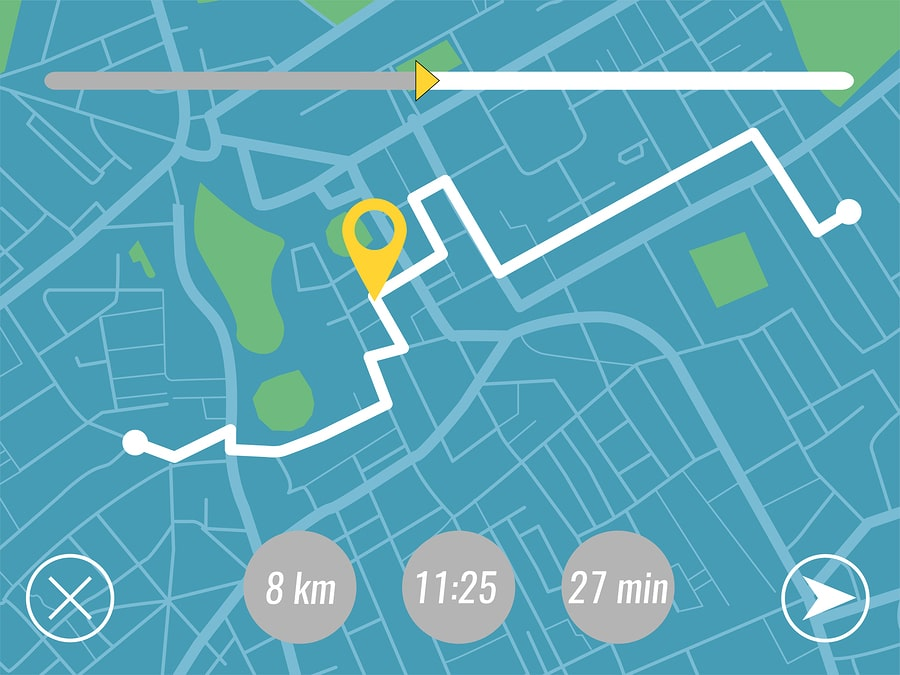## Understanding Distance, Time and Speed

The relationship between distance, time and speed is first covered in primary 6 mathematics. At this time, your child will learn that there are 3 related quantities (distance, time and speed) that are involved in a journey.

In order to comprehend how these 3 relate to each other, we first need to understand what exactly each of these 3 quantities are.

Is your child having difficulty understanding distance, time and speed? If so, consider sending him or her to MLGS’s  primary school maths tuition classes today!

## What is Distance?

Distance is defined as the total movement of an object or how much ground it has covered. We apply our knowledge of distance on a daily basis.

For example, we might make a decision on where to eat at based on its distance from our current position. Alternatively, in realm of sports, soccer players have to gauge the distance between them and their teammates before passing the ball.

### Distance or Displacement?

A distinction needs to be made between distance and a closely related concept – displacement. At first glance, distance and displacement seem to be identical quantities. In fact in many cases, they will produce the same answer.

However, a key difference lies in that displacement takes into account the direction travelled. Since displacement refers to how far out of place an object is, it is possible to have a displacement much lesser than distance.

Two examples demonstrate this:

Firstly, if you were to walk around the edge from one corner to the other, then you would have travelled a distance of:

2m + 4m = 6m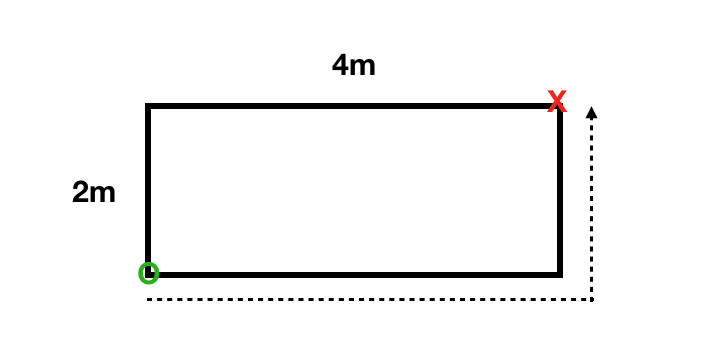However, this is different from displacement as you were displaced diagonally to the other corner of the field. Displacement will then be calculated using the formula for the length of the longest side of a right-angle triangle (also known as a hypotenuse):

(1/2) X 2m X 4m = 4m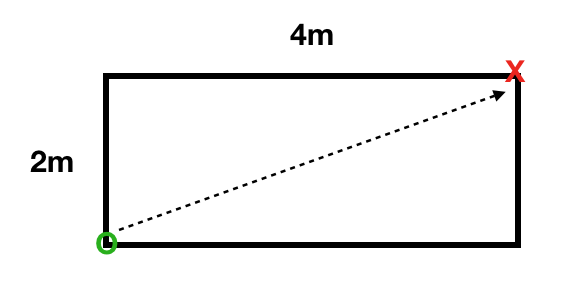Another example would be if you were to walk 5m one direction before reversing, walking past your original point and carrying on forward another 5m.

In this case, you would have travelled a total distance of:

5m + 5m = 10m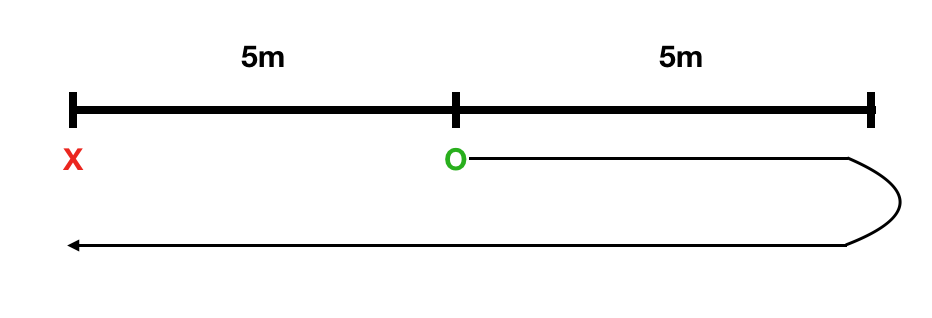This is in contrast with displacement would be:

5m – 5m + 5m = 5m

Essentially, you would only end up 5m away from your original position even though you travelled 10m.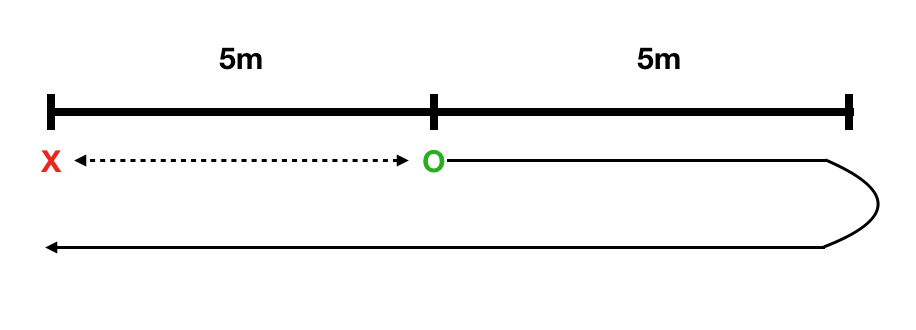## What is Time?

Time is a concept that is familiar to everyone and essential to all of our activities. The uses of time are endless, from allowing us to reliably predict when a sun rises or sets each day, to setting up events in a day.

Like distance, time is also measured in a set of units – seconds, minutes, hours, days, weeks, months and years. Understanding how these units of time work is essential to a host of different activities. For example:

• Finishing your exam paper within the allocated time of an hour
• Setting a 5pm meeting time between you and your friends
• Planning how many days to use for a vacation
• Understanding the changing of seasons in a calendar year

### Time is Objective

Have you ever read phrases such as “time sped up” or “time slowed down”? Technically, these phrases would be incorrect according to the laws of time.

Psychologically, we may feel that time is passing faster or slower depending on our emotions, however time is not actually moving differently at any point. A good objective gauge is then the clock, which reliably proceeds at a uniform pace at all points.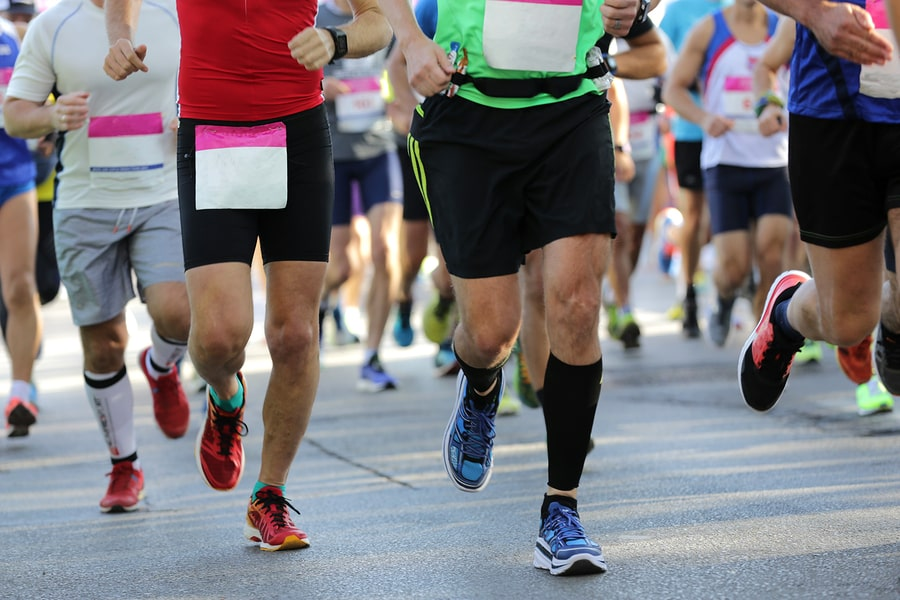## What is Speed?

How fast can you run a certain distance? How quickly can you cook an egg?

These questions relate to the speed of a particular action in relation to time and the action in question. To simplify things, speed can be described as the rate of an action.

Unlike the distance and time, speed is a parameter that is described in relation to other parameters – e.g. distance and time. Often times, by understanding your speed in performing certain action, you will be able to predict the amount of time needed to complete the action.

This brings us to the relationship between distance, time and speed.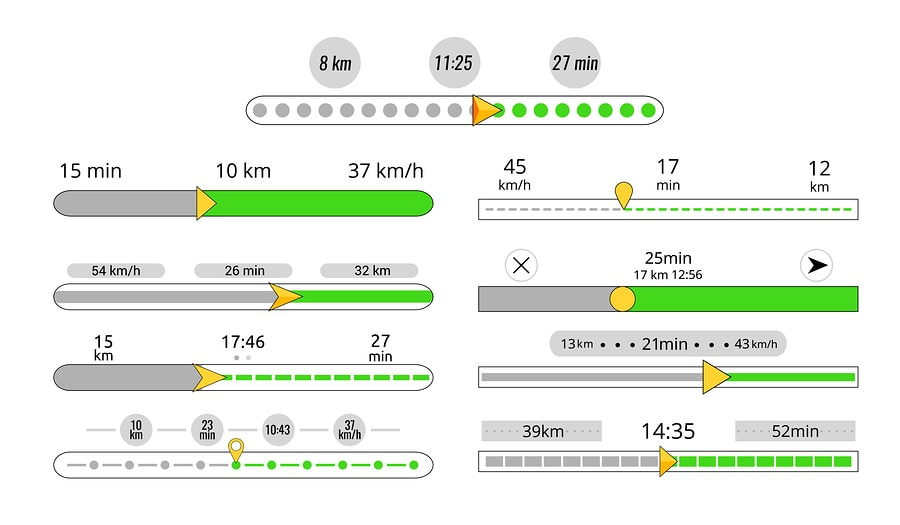## The Relationship Between Distance, Time and Speed

Distance, time and speed are related through the following formula:

Distance = time x speed

To understand this formula, we can incorporate it into a word sentence as follows:

If you were to travel at a speed for a set amount of time, you would have covered a resulting distance.

Putting the formula to use, we are able to work out any one of these properties when we have knowledge of the other two. For example, if you knew the distance between point A and point B, you would be able to determine the speed to travel at given the time targeted.

Alternatively, if you knew the average speed that you could travel at, you could then work out the time needed for the journey.

### Distance, Time and Speed at Primary School Level

In primary school, your child will be introduced to the concepts of distance, time, speed and average speed. To aid in their learning, a technique that is often used is the drawing of a diagram to illustrate the 3 parameters.

Additionally, a challenge that may be thrown in would be the usage of different units of measurement. For example, speed can be illustrated as km/h, m/min or cm/s. This challenges your child’s understanding between different units of measurement.

From this base, your child will be able to apply it to related topics or subjects. For instance, a question may involve working out the time taken for a person to run around a circular stadium. In this case, you would have to apply both formulas for calculating the circumference of a circle as well as distance, time and speed.